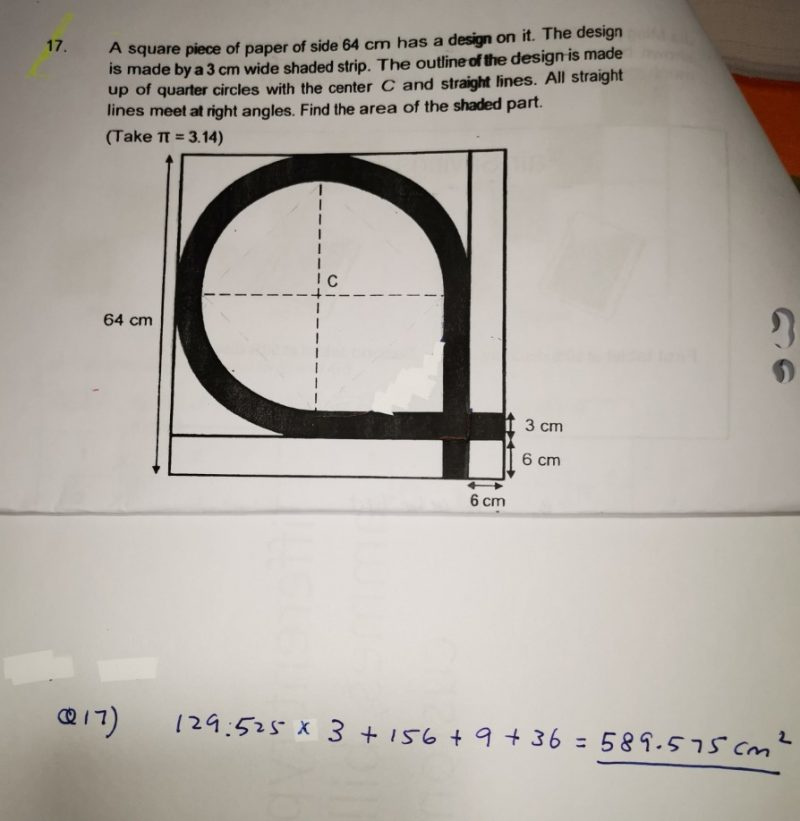QuestionNeed help to answer this question. The answer is there but lack of working.

radius of bigger quarter circles = (64 – 6)/2 = 29
radius of smaller quarter circles = 29 – 3 = 26
area of shaded part = 3 x (1/4) x (3.14) x [(29 x 29) – (26 x 26)] + 2 x (29 + 6) x 3 – (3 x 3) = 388.575 + 201 = 589.575

Ans : 589.575 sq cm.

0 Replies 0 Likes

Radius of big circle –> (64 – 6) ÷ 2 = 29

Area of big circle –> 3.14 x 29 x 29 = 2640.74

Radius of small circle –> 29 – 3 = 26

Area of small circle –> 3.14 x 26 x 26 = 2122.64

Area of the shaded Arc –> ¾ x (2640.74 – 2122.64) = 388.575

Area of the shaded parts formed by straight lines –> 29 x 3 + 26 x 3 + 2 x 6 x 3 = 201

Total Area of Shaded Part –> 201 + 388.575 = 589.575